## C++基础#

### C++对C的扩充#

#### 名字空间#

namespace ns1{
int inflag;
}
namespace ns2{
int inflag;
}

ns1::inflag=2;
ns2::inflag=-3;
// using ns1::inflag; inflag=2;
// ns2::inflag=-3;


#### 用const定义常量#

• 用const定义标识符常量时，一定要对其初始化，这是唯一方法。

#### 引用#

• 对变量起另外一个名字，这个名字称为该变量的引用。

• 其中原变量名必须是一个已定义过的变量。

• 引用并没有重新在内存中开辟存储单元，只是引用原变量的单元。

• 对引用的初始化，可以用一个变量名，也可以用另一个引用。

int   a=3;
int  &b=a;
int  &c=b;

• 引用同变量一样有地址，可以对其地址进行操作，即将其地址赋给一指针。

• 对const常量的引用使用如下方式：

  int i = 5;
const int &ri = i;
// ri = 10;  错误
i = 10;    //正确
cout << ri;

• 以下的声明是非法的

• 企图建立void类型的引用 void &a
• 企图建立引用的数组 int &a
• 企图建立指向引用的指针 int &*p
• 指针和引用的区别：

• 指针是通过地址间接访问某个变量，而引用是通过别名直接访问某个变量。
• 引用必须初始化，而一旦初始化后不再作为其他变量的别名。指针若不进行初始化，编译器不会报错。

#### 引用与函数#

• 函数的返回值为引用类型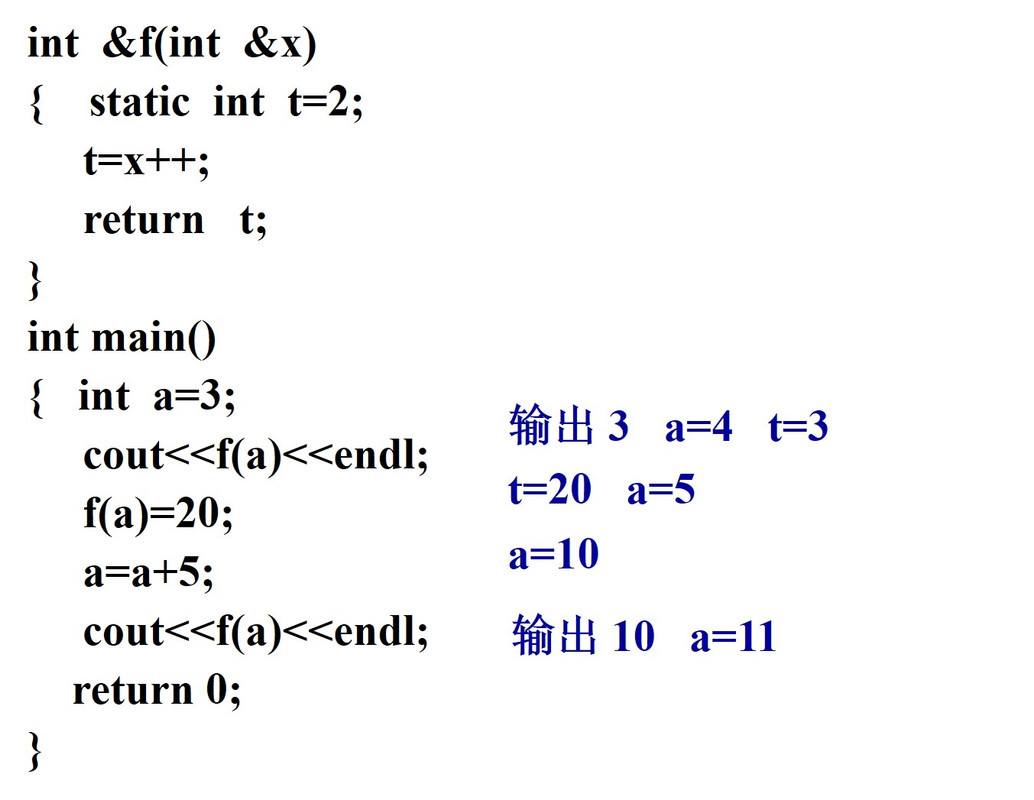#### 内联函数#

• 调用内联函数时，编译器首先检查调用是否正确（类型安全检查或者自动进行类型转换）。如果正确，则将内联函数的代码直接替换函数调用，并用实参换型参，于是省去了函数调用的开销。因此，内联机制增加了空间开销而节约了时间开销。

### 第一次上级题目#

1. 求2个或3个正整数中的最大数，用带有默认参数的函数实现。
2. 输入三个整数，将它们按由小到大的顺序输出，要求使用变量的引用。
3. 编写一个程序，用同一个函数名对几个数据进行从小到大排序，数据类型可以是整型、浮点型。用重载函数实现。
4. 对第3题改用函数模板实现，并与第3题程序进行对比分析。
5. 设计一个日期Date类，它能实现年月日的输入和输出，要求分别将成员函数定义在类体内和类体外。
7. 编写一个基于对象的程序，求出3个长方柱的体积。数据成员包括length(长)、width(宽)、height(高)。要求用成员函数实现以下功能：
(1) 由键盘分别输入3个长方柱的长、宽、高；
(2) 计算长方柱的体积；
(3) 输出3个长方柱的体积。

## 类和对象#

### 复制构造函数调用时机#

1. 程序中需要新建立一个对象，并用另一个同类的对象对它初始化
Box box1(1,2,3);
Box box2 = box1; // Box box2(box1);

1. 当函数的参数为类对象时，在调用函数时需要将实参对象完整的传递给形参
void func(Box b) {
// do something...
}
int main() {
Box box1(1, 2, 3);
fun(box1);
}

1. 当函数的返回值是类的对象，在函数调用完毕将返回值带回函数调用处时
Box f1() {
Box box1(1, 2, 3);
return box1;
}
int main() {
Box box2;
box2 = f();
return 0;
}


### 指向非静态成员函数的指针#

/* Author: fffzlfk */
#include <bits/stdc++.h>
using namespace std;
class Node {
public:
Node(int xx, int yy) : x(xx), y(yy){}
int get_x() { return x; }
int get_y() { return y; }
private:
int x, y;
};

int main() {
Node node(1, 2);
int (Node::*p_x)() = &Node::get_x; // 需要加类名和作用域运算符
int (Node::*p_y)() = &Node::get_y;
cout << (node.*p_x)() << '\n';
cout << (node.*p_y)() << '\n';
return 0;
}


### 指向静态成员函数的指针#

/* Author: fffzlfk */
#include <bits/stdc++.h>
using namespace std;
class A {
public:
static int get() { return 666; }
};

int main() {
int (*p)() = &A::get;
cout << p() << '\n';
cout << (*p)(); // 加不加*都可
return 0;
}


### 对象引用的作用#

Tip

1. 避免通过值来传递对象，而是通过引用来传递
2. 参数传递的是引用，没有构造函数或析构函数被调用，节约了系统资源，提高了运行效率
/* Author: fffzlfk */
#include <bits/stdc++.h>
using namespace std;
class Student {
public:
Student();
Student returnS(Student s) { return s; }
Student(const Student &e) { cout << "Copy Constructor\n"; }
~Student() { cout << "Destructor\n"; }
};
Student::Student() {}
int main() {
Student stu1;
stu1.returnS(stu1);
return 0;
}


Copy Constructor
Copy Constructor
Destructor
Destructor
Destructor

Student& returnS(Student &s) { return s; }


Destructor

### 常对象#

Tip

1. 常对象的所有数据成员都是常量，不能改变。因此，常对象必须初始化。

2. 不能通过常对象调用普通的成员函数，可以调用常成员函数。

3. 如果要修改常对象中某个数据成员的值，可以将数据成员声明为mutable，这样就可以用声明为const的成员函数来修改它的值。

/* Author: fffzlfk */
#include <bits/stdc++.h>
using namespace std;
class A {
private:
mutable int i; // 可以用常成员函数来修改
public:
A() {} //常对象必须初始化
void f_const() const {
i++;
cout << "i = " << i << '\n';
cout << "f_const()" << '\n';
}
void f() {
cout << "f()" << '\n';
}
};
int main() {
const A a; //常对象必须初始化
a.f_const(); //常对象只能调用常成员函数
return 0;
}


#### 常成员#

• 常数据成员

Tip

1. 在任何函数中都不能对常数据成员赋值

2. 只能通过构造函数的参数初始化表对常数据成员进行初始化

const int Hour;
Time::Time(int h) : Hour(h) {}

1. 类的所有对象中的常数据成员的值均不能改变，但不同对象中的该数据成员可以不同（在定义对象时给出）
• 示例程序：
/* Author: fffzlfk */
#include <bits/stdc++.h>
using namespace std;
class A {
public:
A(int i) : a(i) {} // 非静态常数据成员只能通过初始化表来获得初值
void print() {
cout << a << ":" << b << '\n';
}
private:
const int a;
static const int b;
};
const int A::b = 10; // 静态常数据成员只能在类外初始化
int main() {
A a1(100), a2(0);
a1.print();
a2.print();
return 0;
}

• 常成员函数

Tip

1. 通过常成员函数来引用本类中的数据成员，但不能修改他们
2. const 是函数类型的一部分，在声明函数时都要有const，在调用时不必加const
3. 常成员函数不能更新对象的数据成员，也不能调用该类中的非const成员函数
4. 通过常对象只能调用它的常成员函数，而不能调用其他成员函数
5. 常对象中的成员函数不是常成员函数，除非成员函数有const修饰
6. const关键字可以用于对重载函数的区分
/* Author: fffzlfk */
#include <bits/stdc++.h>
using namespace std;
class R {
public:
R(int r1, int r2) {
R1 = r1;
R2 = r2;
}
void print() {
cout << R1 << '-' << R2 << '\n';
}
void print() const {
cout << R1 << "+" << R2 << '\n';
}
private:
int R1, R2;
};

int main() {
R a(5, 4);
a.print(); // 普通对象a调用普通成员函数
const R b(20, 5);
b.print(); // 常对象b调用常成员函数
return 0;
}

• const成员和非const成员之间的调用关系

数据成员非const成员函数const成员函数
非const数据成员可以引用，也可以改变值可以引用，但不可以改变值
const数据成员可以引用，但不能改变值可以引用，但不可以改变值
const对象的数据成员不允许引用和改变值可以引用，不可以改变值

#### const与指针#

• 指向对象的常指针指针本身的值不能改变，即其指向不能改变。

类名 *const 指针变量名 = 对象地址

Time t1(10, 12, 15), t2;
Time *const ptr1 = &t1;
ptr1 = &t2; //错误，ptr1不能改变指向


Tip

常指针始终指向同一个对象，但是可以改变其所指对象中数据成员的值。

• 指向常对象的指针

const 类名 *指针变量名 = 对象地址

• 如果存在一个常对象，只能用指向常对象的指针去指向它，而不能用非const型的指针去指向它。

• 指向常对象的指针还可以指向非const型的对象，此时不能通过指针改变该对象的值，但是通过该对象本身来改变。指针本身的值也可以改变。

Time t1(10, 12, 15), t2;
const Time *p = &t1; // p是指向常对象的指针，并指向t1对象
(*p).hour = 18;      // 错误，不能通过指针改变t1的值
t1.hour = 18;        // 正确，t1不是常对象
p = &t2;             // 正确，p改为指向t2


Note

指向常对象的指针可以指向const和非const型的对象，而指向非const型对象的指针只能指向非const的对象。

### 对象数组#

/* Author: fffzlfk */
#include <bits/stdc++.h>
using namespace std;
class Box {
public:
Box(int h = 10, int w = 12, int len = 15)
: height(h), width(w), length(len) {}
int volume();

private:
int height, width, length;
};

int Box::volume() { return height * width * length; }

int main() {
Box a{Box(10, 12, 15),
Box(15, 18, 20)};  // Box a = {Box(10, 12, 15), Box(15, 18,
// 20)}; 加不加等号都可
cout << a.volume() << '\n';
cout << a.volume() << '\n';
}


### 类模板#

#### 类模板中的成员函数的定义#

1. 可以放在类模板的定义定义体中（此时与类中的成员函数定义方法一致）

2. 也可以放在类模板的外部，此时成员函数的定义格式如下：

template<class 类型参数>
<返回值类型> <类模板名><类型参数>::<函数名> (<参数表>) {
<函数体>
}


template <class 类型参数>

### 第二次上机题目#

1. 编写设计一个People（人）类。该类的数据成员有年龄（age）、身高（height）、体重（weight）和人数（num），其中人数为静态数据成员，成员函数有构造函数（People）、进食（Eating）、运动（Sporting）、睡眠（Sleeping）、显示（Show）和显示人数（ShowNum）。其中构造函数由已知参数年龄(a)、身高(h)和体重(w)构造对象，进食函数使体重加1，运动函数使身高加1，睡眠函数使年龄、身高、体重各加1，显示函数用于显示人的年龄、身高、体重，显示人数函数为静态成员函数，用于显示人的个数。假设年龄的单位为岁，身高的单位为厘米，体重的单位为市斤，要求所有数据成员为protected访问权限，所有成员函数为public访问权限，在主函数中通过对象直接访问类的所有成员函数。
2. 定义一个描述学生（Student）基本情况的类，数据成员包括姓名(name)、学号(num)、数学成绩(mathScore)、英语成绩(englishScore)、人数(count)、数学总成绩(mathTotalScore)和英语总成绩(englishTotalScore)。其中姓名定义为长度为18的字符数组，其他数据成员类型为整型，数学总成绩、英语总成绩和人数为静态数据成员，函数成员包括构造函数、显示基本数据函数（ShowBase）和显示静态数据函数(showStatic)，其中构造函数由已知参数姓名(nm)、学号(nu)、数学成绩(math)和英语成绩(english)构造对象，显示基本数据函数用于显示学生的姓名、学号、数学成绩、英语成绩，显示静态数据函数为静态成员函数，用于显示人数、数学总成绩、英语总成绩；要求所有数据成员为private访问权限，所有成员函数为public访问权限，在主函数中定义若干个学生对象，分别显示学生基本信息，以及显示学生人数，数学总成绩与英语总成绩。
3. 定义一个Dog，包含name、age、sex和weight等属性以及对这些属性操作的方法。要求用字符指针描述name，并且用对象指针来测试这个类。
4. 管理个人活期账户：个人储蓄活期账户包括账号、户名、密码、余额、活期年利率等信息。要求能够对个人账户进行存钱、取钱、计算年利息、打印账户相关信息等操作。编写主函数测试账户相关功能。 5．建立一个对象数组，内放5个学生的数据（学号、成绩），（1）用指针指向数组首元素，输出第1，3，5个学生的数据；（2）设立一个函数max，用指向对象的指针作函数参数，在max函数中找出5个学生中成绩最高者，并输出其学号。

## 运算符重载#

::作用域运算符
.成员访问运算符
.*成员指针
?:条件运算符
sizeof长度运算符

### 重载为类的成员函数#

<函数类型> operator <运算符>(<参数表>) {函数体}

• C++中不允许重载有三个操作数的运算符
• 运算符作为成员函数时最多有一个形参：参数可以是对象，对象的引用，或其它类型的参数
• 运算符重载的实质就是函数重载
• 运算符重载的函数参数就是该运算符涉及的操作数

#### 单目运算符的重载++、–#

• ++为前置运算符时： operator++() {…}
• ++为后置运算符时： operator++(int) {…}

### 友元函数#

Tip

• 友元函数是可以直接访问类的私有成员的非成员函数。
• 它是定义在类外的普通函数，它不属于任何类，但需要在类的定义中加以声明，声明时只需在友元的名称前加上关键字friend，其格式如下：
friend 类型 函数名(形式参数);
#include <bits/stdc++.h>
using namespace std;
class A {
int i;

public:
friend void foo(A);
};
void foo(A a) { cout << a.i << '\n'; }

int main() {
A a;
foo(a);
}

• 大多数情况下友元函数是某个类的成员函数，即A类中的某个成员函数是B类中的友元函数，这个成员函数可以直接访问B类中的私有数据。这实现了类与类之间的沟通。

class A {               class B {
void fun(B &);          friend void A::fun(B &b);
};                      };

• 友元类

class A {             class B {
...                   ...
// B类是A类的友元       // B类可以自由使用A类中的成员
friend class B;     }；
};


### 重载为类的友元函数#

friend <函数值类型> operator<运算符>(<参数表>) {函数体}

• 运算符重载为类的友元函数****最多只能有两个参数**
• 如果重载双目运算符，则第一个参数代表左操作数，第二个参数代表右操作数

#### 单目运算符重载#

• ++为前置运算符时，它的运算符重载函数的一般格式为：A operator ++(A &a)
• ++为后置运算符时，它的运算符重载函数的一般格式为：A operator ++(A &a, int)(使用哑元区分)

### 重载输入输出运算符#

• 输入运算符：friend istream & operater »(istream &is, ClassName &f){…}

• 输出运算符：friend ostream & operater «(ostream &, ClassName &){…}

### 函数对象#

#include <bits/stdc++.h>
using namespace std;
class Test {
public:
int operator()(int a, int b) {
cout << "operator() called. " << a << ' ' << b << endl;
return a + b;
}
};

int main() {
Test sum;
int s = sum(3, 4);  // sum看上去像是一个函数，故也称“函数对象”
cout << "a + b = " << s << endl;
}


### 类型转换运算符重载#

#### 基本类型到类类型的转换#

• 如果直接将数据赋值给对象，所赋入的数据要强制类型转换，这种转换需要调用构造函数。也就是利用构造函数能完成基本类型到类类型的转换
• 使用构造函数进行类型转换必须有一个前提，那就是在这个类中定义一个只有一个参数的构造函数（或者其他参数有默认值）——转换构造函数

#### 类类型到基本类型的转换#

• C++引入一种特殊的成员函数——类型转换函数。类型转换函数实际上就是一个类型转换运算符重载函数

• 类型转换函数专门用来将类类型转换为基本数据类型，它只能被重载为成员函数

• 重载类型转换运算符函数格式：
operator〈返回基本类型名〉（） { …… return 〈基本类型值〉 }

#include <bits/stdc++.h>
using namespace std;
class A {
int i;

public:
A(int a = 0) : i(a) {}
void show(void) { cout << "i = " << i << '\n'; }
operator int() { return i; }
};

int main() {
A a1(10), a2(20);
cout << a1 << '\n';
cout << a2 << '\n';
return 0;
}


### 示例代码#

#include <bits/stdc++.h>
using namespace std;

class Complex {
double real, imag;

public:
Complex(double r = 0.0, double i = 0.0) : real(r), imag(i) {}
double getReal() const { return real; }
double getImag() const { return imag; }
Complex operator++();
Complex operator++(int);
Complex operator+(Complex &);
Complex operator+(double d);
friend istream &operator>>(istream &, Complex &);
friend ostream &operator<<(ostream &, const Complex &);
};

Complex Complex::operator++() { return Complex(++real, imag); }

Complex Complex::operator++(int a) { return Complex(real++, imag); }

Complex Complex::operator+(Complex &c) {
return Complex(real + c.real, imag + c.imag);
}

Complex Complex::operator+(double d) { return Complex(real + d, imag); }

istream &operator>>(istream &in, Complex &c) {
in >> c.real >> c.imag;
return in;
}

ostream &operator<<(ostream &out, const Complex &c) {
out << to_string(c.real) + "+" + to_string(c.imag) + "i";
return out;
}

int main() {
Complex a(1, 2);
cout << a + a << '\n';
cout << a++ << '\n' << ++a << '\n';
a = 10;  // 相当于a = Complex(10); 产生临时对象，调用构造函数和析构函数
cout << a << '\n';
return 0;
}


## 继承与派生#

### 派生类的构造函数和析构函数#

#### 派生类构造函数#

• 不能在派生类构造函数体中显式调用基类构造函数

• 在成员初始化表中可以显式调用基类构造函数

Rectangle(float x,float y,float w,float h) : Point(x,y)
{ W=w; H=h; }
或:
Rectangle(float x,float y,float w,float h) : Point(x,y),W(w),H(h) {}


#### 构造函数和析构函数的调用顺序#

• 构造函数调用顺序：基类的构造函数->对象成员构造函数->派生类构造函数

• 析构函数调用顺序刚好相反

#include <bits/stdc++.h>
using namespace std;
class O {
public:
O() { cout << "O()" << '\n'; }
~O() { cout << "~O()" << '\n'; }
};

class A {
public:
A() { cout << "A()" << '\n'; }
~A() { cout << "~A()" << '\n'; }
};

class B : public A {
O o;

public:
B() { cout << "B()" << '\n'; }
~B() { cout << "~B()" << '\n'; }
};
int main() {
B b;
return 0;
}

A()
O()
B()
~B()
~O()
~A()

• ⚠️注意

• 当基类中没有显式定义构造函数，或定义了无参数构造函数时，派生类构造函数的初始化表可以省略对基类构造函数的调用，而采用隐含调用
• 当基类的构造函数使用一个或多个参数时候派生类必须定义构造函数，提供将参数传递给基类的构造函数的途径。这时，派生类构造函数体可能为空，仅起到参数传递作用
• 无论是哪种继承方式，基类的私有成员在派生类中都是不可被访问的。只能通过基类的成员函数访问基类的私有数据成员。
• 如果在一个派生类中要访问基类中的私有成员，可以将这个派生类声明为基类的友元。
class Base {              class Derive : public Base {
friend class Derive;        // 直接使用Base中的私有成员
}                         }

• 友元关系是不能继承的：B类是A类的友元，C类是B类的派生类，则C类和A类之间没有任何友元关系，除非C类声明A类是友元。

### 多继承与虚基类#

#### 多继承派生类的定义#

class <派生类名>：<继承方式> <基类名1>，…，<继承方式> <基类名n>
{
<派生类新定义成员>
}；


#### 多继承派生类的构造函数#

<派生类名>(<总参数表>):<基类名1>(<参数表1>)，…，< 基类名n> (<参数表n>)
{
<派生类数据成员的初始化>
};

• 构造函数的调用顺序是：先调用所有基类的构造函数，再调用对象成员构造函数，最后调用派生类的构造函数

• 处于同一层次的各基类构造函数的调用顺序取决于定义派生类时所指定的基类顺序，与派生类构造函数中所定义的成员初始化列表无关

• 如果有多个成员类对象，则构造函数额调用顺序是对象在类中被声明的顺序，而不是它们出现在成员初始化列表的顺序

• 析构函数的调用顺序与构造函数的调用顺序相反

#include <bits/stdc++.h>
using namespace std;

class M1 {
int i;

public:
M1(int _i) : i(_i) { cout << "M1(" << i <<  ")" << '\n'; }
};

class M2 {
int i;

public:
M2(int _i) : i(_i) { cout << "M2(" << i <<  ")" << '\n'; }
};

class Base1 {
public:
Base1() { cout << "Base1()" << '\n'; }
};

class Base2 {
public:
Base2() { cout << "Base2()" << '\n'; }
};

class Derive : public Base1, public Base2 {
M2 m2;
M1 m1;

public:
Derive(int a, int b) : m1(a), m2(b), Base2()  , Base1() {}
};

int main() {
Derive d(8, 9);
return 0;
}

Base1()
Base2()
M2(9)
M1(8)


### 多继承引起的二义性问题#

#### 两个基类有同名成员#

• implementation
#include <bits/stdc++.h>
using namespace std;
class A {
public:
void display() { cout << "display() in A"   << '\n'; }
};

class B {
public:
void display() { cout << "display() in B"   << '\n'; }
};

class C : public A, public B {

};

int main() {
C c;
c.display();
return 0;
}

• 编译错误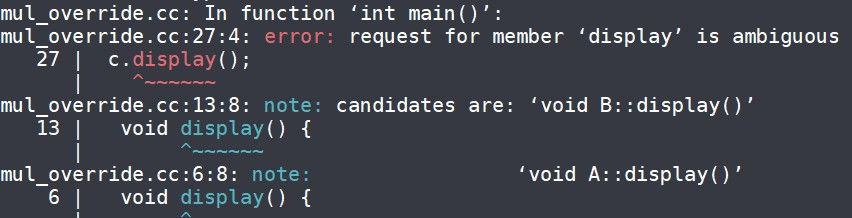#### 两个基类和派生类三者都有同名成员#

• 基类的同名成员在派生类中被屏蔽，或者说，派生类新增加的同名成员隐藏了基类中的同名成员
• implementation
#include <bits/stdc++.h>
using namespace std;
class A {
public:
void display() { cout << "display() in A"   << '\n'; }
};

class B {
public:
void display() { cout << "display() in B"   << '\n'; }
};

class C : public A, public B {
public:
void display() { cout << "display() in C"   << '\n'; }
};

int main() {
C c;
c.display();
return 0;
}

• 访问的是派生类C中的成员
display() in C


### 虚基类#

#### 虚基类概念#

• 为了避免对基类成员访问的二义性问题，可以将直接基类（如A、B）的共同基类如N设置为虚基类，这样共同基类N在内存中只有一个副本存在
• 引进虚基类后，派生类对象中只存在一个虚基类成员的副本
• 为了保证虚基类在派生类中只继承一次，应当在该基类的所有直接派生类中声明为虚基类，否则仍然会出现对基类的多次继承。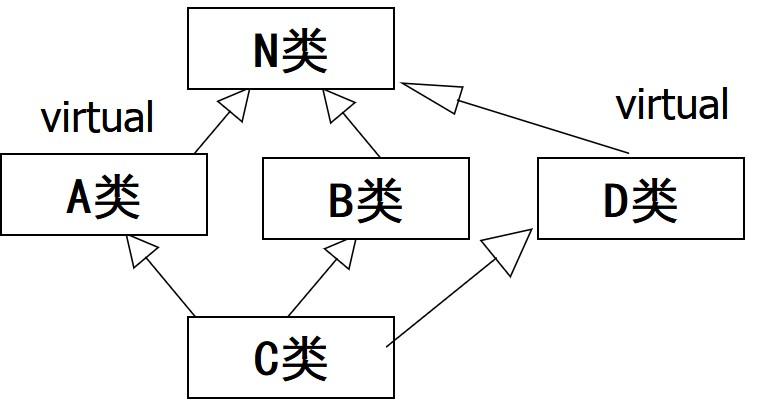#### 构造函数的调用顺序#

• 先调用虚基类的构造函数，再调用非虚基类的构造函数
• 若同一层次包含多个虚基类，其调用顺序为定义时的顺序
• 若虚基类构造函数由非虚基类派生而来，则仍先按先调用基类构造函数，再调用派生类构造函数的顺序
#include <bits/stdc++.h>
using namespace std;

class Base1 {
public:
Base1() { cout << "class Base1" << endl; }
};
class Base2 {
public:
Base2() { cout << "class Base2" << endl; }
};
class Level1 : public Base2, virtual public   Base1 {
public:
Level1() { cout << "class Level1" << endl; }
};
class Level2 : public Base2, virtual public   Base1 {
public:
Level2() { cout << "class Level2" << endl; }
};
class TopLevel : public Level1, virtual public  Level2 {
public:
TopLevel() { cout << "class TopLevel" <<  endl; }
};
int main() {
TopLevel obj;
return 0;
}

输出
class Base1
class Base2
class Level2
class Base2
class Level1
class TopLevel


#### 虚基类的初始化#

class A
{ A(int i){} … };

class B:virtual public A
{ B(int n):A(n){}… };

class C:virtual public A
{ C(int n):A(n){}… };

class D:public B, public C
{ D(int n):A(n),B(n),C(n){}… };

• ⚠️注意
• 如果多继承不牵扯到对同一基类的派生，就没有必要定义虚基类
• 使用多继承要十分小心，经常会出现二义性问题
• 能用单一继承的问题就不要用多继承

## 多态性与虚函数#

### 类型兼容规则#

• 派生类的对象可以赋值给基类对象
• 派生类的对象可以初始化基类的引用
• 派生类的对象的地址可以赋给基类的指针变量
#include <bits/stdc++.h>
using namespace std;
class Base {
public:
void who() { cout << "Base class" << endl; }
};

class Derive1 : public Base {
public:
void who() { cout << "Derive1 class" << endl; }
};

class Derive2 : public Base {
public:
void who() { cout << "Derive2 class" << endl; }
};

int main() {
Base obj1, *p;
//定义基类对象obj1和基类对象指针p
Derive1 obj2;
Derive2 obj3;
p = &obj1;  // p指向obj1
p->who();
//通过指针p调用obj1的公有成员函数//who()
p = &obj2;  // p指向obj2
p->who();
// p只能访问从基类继承下来的who()
p = &obj3;
// p指向obj2
p->who();
// p只能访问从基类继承下来的who()
obj2.who();
obj3.who();
return 0;
}


Base class
Base class
Base class
Derive1 class
Derive2 class

#include <bits/stdc++.h>
using namespace std;

class Student {
int num;

public:
Student(int n) : num(n) {}
void display() { cout << "num = " << num << endl; }
};

class Graduate : public Student {
string name;

public:
Graduate(int n, string _name) : Student(n), name(_name) {}
void display() {
Student::display();
cout << "name = " << name << '\n';
}
};

int main() {
Student stu(1001);
Student *pt = &stu;
pt->display();
pt->display();
return 0;
}


num = 1001
num = 2001


### 多态（Polymorphism）#

#### 多态的分类#

• 静态多态性（编译时的多态性）：通过函数重载实现
• 动态多态性（运行时的多态性）：通过虚函数实现

#### 虚函数#

virtual <函数值类型> <函数名>(<参数表>);

• 程序运行时，不同类的对象调用各自的虚函数，这就是动态多态

• 实现动态的多态性时，必须使用基类类型的指针变量或对象引用，并使其指向不同的派生对象，并通过调用指针或引用所指向的虚函数才能实现动态的多态性

• 声明虚函数要注意

• 静态成员函数和友元函数不能声明为虚函数
• 内联成员函数不能声明为虚函数
• 构造函数不能是虚函数
• 析构函数可以是虚函数
#include <iostream>
using namespace std;
class Base {
public:
Base() = default;
virtual ~Base() { cout << "Base destructor" << '\n'; }
};

class Derived : public Base {
public:
Derived() = default;
~Derived() { cout << "Derived destructor" << '\n'; }
};

int main() {
Base *b = new Derived;
delete b;
return 0;
}


#### 联编#

• 联编的分类
• 静态联编：在编译阶段完成的联编

#include <iostream>
using namespace std;
class Student {
public:
void print() { cout << "A student" << endl; }
};
class GStudent : public Student {
public:
void print() { cout << "A graduate student" << endl; }
};
int main() {
Student s1, *ps;
GStudent s2;
s1.print();
s2.print();
s2.Student::print();
ps = &s1;
ps->print();  //基类指针和基类成员函数发生关联
ps = &s2;
ps->print();
//希望调用对象s2的输出函数，但调用的却是对象s1的输出函数
return 0;
}


输出

A student
A student
A student
A student

• 动态联编：根据具体的执行情况来动态的确定，在运行阶段完成

#include <bits/stdc++.h>
using namespace std;
class Student {
public:
// virtual可省略
virtual void print() { cout << "a Student" << '\n'; }
};

class GStudent : public Student {
public:
virtual void print() { cout << "A graduate student" << '\n'; }
};
int main() {
Student s1, *ps;
GStudent s2;
s1.print();
s2.print();
s2.Student::print();
ps = &s1;
ps->print();
ps = &s2;
// 对象指针调用虚函数，采用动态联编方式
ps->print();
return 0;
}


输出

a Student
a Student
a Student

#include <bits/stdc++.h>
using namespace std;
class Student {
public:
virtual void print() { cout << "A studnet" << '\n'; }
};

class GStudent : public Student {
public:
void print() { cout << "A graduate studnet" << '\n'; }
};

void fun(Student &s) { s.print(); }
int main() {
Student s1;
GStudent s2;
fun(s1);
fun(s2);
return 0;
}


输出

A studnet

• ⚠️注意
• virtual关键字只能用在虚函数声明中，不能用在虚函数的实现中
• 只有通过对象指针或对象引用来调用虚函数，才能实现动态联编。如果采用对象来调用虚函数，则采用的是静态联编方式
• 在派生类中重新定义虚函数时，返回值类型、函数名、参数个数、类型和顺序，都必须与基类的原型相同
• 当在派生类中定义了虚函数的重载函数，但并没有重新定义虚函数时，与虚函数同名的重载函数覆盖了派生类中的虚函数。此时若试图通过派生类对象、指针调用派生类对象的虚函数就会产生错误
#include <bits/stdc++.h>
using namespace std;

const int PI = 3.1415;
class Point {
int X, Y;

public:
Point(int X = 0, int Y = 0) {
this->X = X;
this->Y = Y;
}
virtual double area() { return 0.0; }
};

class Circle : public Point {

public:
Circle(int X, int Y, double R) : Point(X, Y) { radius = R; }
};

int main() {
Point P1(10, 10);
cout << "P1.area = " << P1.area() << endl;
Circle C1(10, 10, 20);
cout << "C1.area = " << C1.area() << endl;
Point *Pp;
Pp = &C1;
cout << "Pp.area = " << Pp->area() << endl;
Point &Rp = C1;
cout << "Rp.area = " << Rp.area() << endl;
return 0;
}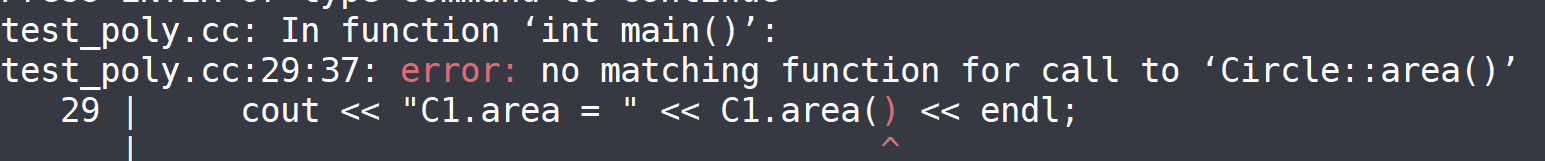• 如果在派生类中没有重新定义虚函数，则不实现动态联编，派生类的对象将使用基类虚函数的代码
• 一个类中的虚函数说明只对派生类中重定义的函数有影响，对它的基类中的函数并没有影响
#include <bits/stdc++.h>
using namespace std;
class Base {
public:
int func(int x) {
cout << "This is Base class" << endl;
return x;
}
};

class SubClass : public Base {
public:
virtual int func(int x) {
cout << "This is SubClass" << endl;
return x;
}
};
class SubSubClass : public SubClass {
public:
int func(int x) {
cout << "This is SubSubClass" << endl;
return x;
}
};
int main() {
SubSubClass ss;
Base &b = ss;
cout << b.func(5) << endl;
SubClass &s = ss;
cout << s.func(5) << endl;
return 0;
}

输出
This is Base class  5
This is Sub2 class  5


#### 纯虚函数#

• 纯虚函数与函数体为空的虚函数

• 区别
• 前者没有函数体，后者有函数体
• 前者所在的类是抽象类，不能直接实例化；后者所在的类是可以实例化的（该类中不含有其他纯虚函数）
• 共同点
• 可以派生出新的类，然后在新类中给出虚函数的实现，而且这种实现可以具有动态特征
#include <bits/stdc++.h>
using namespace std;
class A {
protected:
int x;

public:
A() { x = 1000; }
virtual void print() = 0;  // 定义纯虚函数
};

class B : public A {
int y;

public:
B() { y = 2000; }
void print() { cout << "y = " << y << '\n'; }  // 重新定义纯虚函数
};

class C : public A {
int z;

public:
C() { z = 3000; }
void print() { cout << "z = " << z << '\n'; }  // 重新定义纯虚函数
};

int main() {
A *pa;
B b;
C c;
pa = &b;
pa->print();
pa = &c;
pa->print();
// A a;          不能定义抽象类的对象
// pa = &a;
// pa->pritn();
return 0;
}


输出

y = 2000
z = 3000


#### 抽象类#

• 包含一个或多个纯虚函数的类称为抽象类

• 如果派生类没有实现基类中的所有纯虚函数，派生类也是抽象类

• 抽象类无法实例化

• 抽象类不能用作参数类型、函数值类型或显式转换的类型，但可以声明指向抽象类的的指针或引用，通过指针或引用来指向并访问派生类对象，从而实现动态多态

#include <bits/stdc++.h>
using namespace std;
const double PI = 3.14159;
class Shapes  //抽象类
{
public:
void setvalue(int d, int w = 0) {
x = d;
y = w;
}
virtual void area() = 0;  //纯虚函数
protected:
int x, y;
};
class Square : public Shapes {
public:
void area()  //计算矩形面积
{
cout << "area of rectangle:" << x * y << endl;
}
};
class Circle : public Shapes {
public:
void area()  //计算圆面积
{
cout << "area of circle:" << PI * x * x << endl;
}
};
int main() {
Shapes *ptr;  //声明抽象类指针
Square s1;       //声明派生类对象
Circle c1;       //声明派生类对象
ptr = &s1;
//抽象类指针指向派生类对象
ptr->setvalue(10, 5);
ptr->area();
//抽象类指针调用派生类成员函数,实现多态
ptr = &c1;
//抽象类指针指向派生类对象
ptr->setvalue(10);
ptr->area();
//抽象类指针调用派生类成员函数,实现多态
return 0;
}


输出

area of rectangle:50
area of circle:314.159


### 第三次上机题目#

1. 定义一个复数类Complex，重载运算符“+”，“-”，“*”，“/”,使之能用于复数的加、减、乘、除。运算符重载函数作为Complex类的成员函数。编程序，分别求两个复数之和、差、积和商。
2. 对于2行3列矩阵，重载流插入运算符“«”和流提取运算符“»”，使之能用于矩阵的输入和输出。
3. 定义Time类和Date类，Time类为Date类的友元类，通过Time类中的display函数引用Date类对象的私有数据，输出年、月、日和时、分、秒。
• 在两个基类中都包含姓名、年龄、性别、地址、电话等数据成员。
• 对两个基类中的姓名、年龄、性别、地址、电话等数据成员用相同的名字，在引用这些数据成员时，指定作用域。
• 在类体中声明成员函数，在类外定义成员函数。
5. 写一个程序，定义抽象基类Shape，由它派生出5个派生类：Circle（圆形）、Square（正方形）、Rectangle（矩形）、Trapezoid（梯形）、Triangle（三角形）。用虚函数分别计算几种图形面积，并求它们的和。要求用基类指针数组，使它的每一个元素指向一个派生类对象。

## 输入输出流#

### I/O流类库的层次结构#

• C++编译系统提供的I/O流类库含有两个平行基类：
• streambuf
• ios
• ios类有4个直接派生类：
• 输入流类istream
• 输出流类ostream
• 文件流类基类fstreambase
• 字符串流类基类strstreambase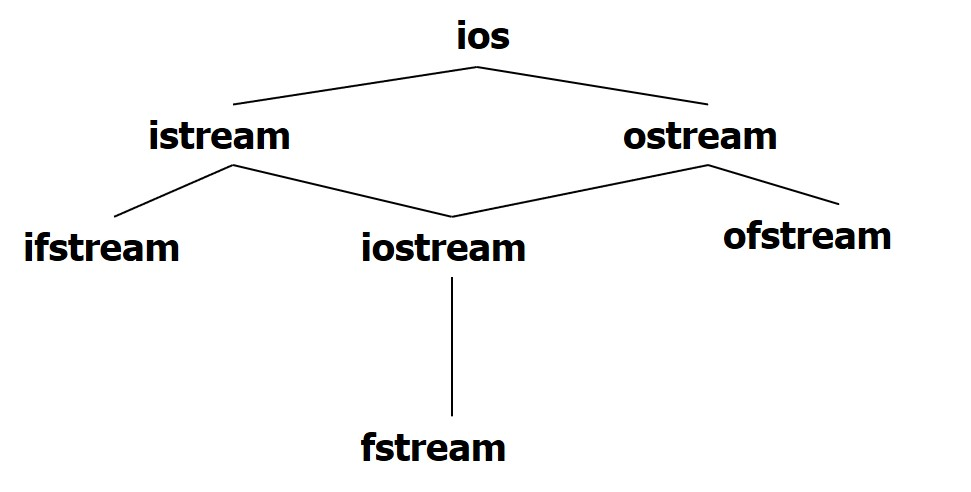### I/O流类库的头文件#

• iostream：I/O流类库的最主要的头文件，包含了对输入输出流进行操作的所需的基本信息，还包括cin、cout、cerr、clog共4个流对象
• fstream：用于用户管理的文件的I/O操作
• strstream：用于字符串流I/O
• stdiostream：用于混合使用C和C++的I/O操作
• iomanip：用于格式化I/O时应包含此头文件

### 输入输出的格式控制#

• 两种格式化方式
• 用流对象的有关成员函数进行格式化
• 用专门的控制符进行格式化输入输出

#### 用流对象的成员函数格式化#

• 设置状态标志
long ios::setf(long flags)

• 清楚状态标志
long ios::unsetf(long flags)

• 取状态标志
long ios::flags()

• 取状态标志并设置状态标志
long ios::flags(long flag)


⚠️以上三组函数必须用流式对象（cin或cout）来调用

#include <bits/stdc++.h>
using namespace std;
void showflags(long f) {
long i;
for (i = 0x8000; i; i >>= 1) {
cout << ((i & f) ? "1" : "0");
}
cout << '\n';
}

int main() {
long f;
f = cout.flags();
showflags(f);
cout.setf(ios::showpos | ios::scientific | ios::fixed);  // 追加状态标志
f = cout.flags();
showflags(f);
cout.unsetf(ios::scientific);  // 从状态标志中去掉scientific
f = cout.flags();
showflags(f);
f = cout.flags(ios::hex);  // 重新设置状态标志
showflags(f);              // 重新设置状态标志之前
f = cout.flags();
showflags(f);
return 0;
}

• 用流对象的成员函数设置输出宽度
int ios::width(int len)
int ios::width()

• 第一种设置输出宽度并返回原来的输出宽度；第二种返回当前输出宽度，默认输出宽度为0
• 只对其后的第一个输出项有效
• 设置填充字符
char ios::fill(char ch)
char ios::fill()

• 设置输出精度
int ios::precision(int p)
int ios::precision()

• 默认输出精度为6
• 用流成员函数put输出字符
cout.out('a')

#include <bits/stdc++.h>
using namespace std;

int main() {
int i = cout.width();
cout << "width: " << i << endl;
cout.width(8);
cout << cout.width() << "new width" << endl;
char c = cout.fill();
cout << "filling word is: " << c << "(ASCII code" << (int)c << ")" << endl;
cout.fill('*');
cout << cout.fill() << "(" << (int)cout.fill() << ")(new filling word)"
<< endl;
int j = cout.precision();
cout << "precision: " << j << endl;
cout.precision(8);
cout << 123.456789 << "(example)" << endl;
cout << cout.precision() << "(new precision)" << endl;
return 0;
}

width:0
8(new width)
filling word is: (ASCII code32)
*(42)(new filling word)
precision:6
123.45679(example)
8(new precision)

• 用于字符输入的流成员函数
• 不带参数的get函数
#include <bits/stdc++.h>
using namespace std;

int main() {
int c;
cout << "enter a sentence:" << endl;
while ((c = cin.get()) != EOF) // 函数的返回值就是读入的字符
cout.put(c);
return 0;
}

• 有一个参数的get函数
#include <bits/stdc++.h>
using namespace std;

int main() {
char c;
cout << "enter a sentence:" << endl;
while (cin.get(
c))  // 读取一个字符赋给c，如果读取成功，返回真，如失败（如遇文件结束符）则返回假
cout.put(c);
cout << "end" << endl;
return 0;
}

• 有三个参数的get函数
• cin.get(字符数组/字符指针, 字符个数n, 终止字符)
• 从输入流中读取n-1个字符，赋给指定的字符数组（或字符指针指向的数组）
• 如果在读取n-1个字符之前遇到指定的终止字符，则提前读取结束
• 如果读取成功返回真，失败（遇文件结束符）则返回假
#include <bits/stdc++.h>
using namespace std;

int main() {
char ch;
cout << "enter a sentence:" << endl;
cin.get(ch, 10, '/');
cout << ch << endl;
cin.get(ch, 20, '\n');  // cin.get(ch, 20);
cout << ch << endl;
return 0;
}

enter a sentence:
you!/her.
you!
/her.

• 用getline函数读入一行字符
• cin.getline(字符数组（或字符指针）, 字符个数n, 终止字符)
• 从输入流中读取一行字符，其用法与带3个参数的get函数类似
#include <bits/stdc++.h>
using namespace std;

int main() {
char ch;
cout << "enter a sentence:" << endl;
cin >> ch;
cout << ch << endl;
cin.getline(ch, 20, '/');
cout << ch << endl;
cin.getline(ch, 20);
cout << ch << endl;
return 0;
}

enter a sentence:
I like C++./I study C++./I am happy.
I
like C++.
I study C++./I am h

• eof函数
while(!cin.eof())

• peek函数
• 无参函数，表示“观察”，观测下一字符
• 返回值是当前指针指向的当前字符，但只是观测，指针仍停留在当前位置，并不后移
• 如果要访问的字符是文件结束符时，则函数值是EOF
c = cin.peek();

• putback函数
• 将前面用get或getline函数从输入流中读取的字符ch返回到输入流，插入到当前指针位置，供后面读取
#include <bits/stdc++.h>
using namespace std;

int main() {
char c;
int ch;
cout << "enter a sentence:" << endl;
cin.getline(c, 15, '/');
cout << c << endl;
ch = cin.peek(); // 观看当前字符
cout << ch << endl;
cin.putback(c); // 将'I'插入到指针所指处
cin.getline(c, 15, '/');
cout << c << endl;
return 0;
}

enter a sentence:
I am a boy./ am a student./
I am a boy.
32
I am a student

• ignore函数
• cin.ignore(n, 终止字符)
• 跳过输入流中n个字符，或在遇到指定的终止字符时提前结束（此时跳过包括终止字符在内的若干字符）
ignore(5, 'A'); // 跳过5个字符，遇'A'后不再跳
ignore(); <-> ignore(1, EOF);

• 示例代码
• 不加ignore
#include <bits/stdc++.h>
using namespace std;

int main() {
char ch;
cin.get(ch, 20, '/');
cout << "the first part is: " << ch << endl;
cin.get(ch, 20, '/');  // get不跳过终止字符
cout << "the second part is: " << ch << endl;
return 0;
}

I like C++./I study C++./I am happy.
The first part is:I like C++.
The second part is:

• 加ignore
#include <bits/stdc++.h>
using namespace std;

int main() {
char ch;
cin.get(ch, 20, '/');
cout << "the first part is: " << ch << endl;
cin.ignore();          // 跳过输入流中一个字符
cin.get(ch, 20, '/');  // get不跳过终止字符
cout << "the second part is: " << ch << endl;
return 0;
}

I like C++./I study C++./I am happy.
The first part is:I like C++.
The second part is:I study C++.

• 用控制符格式化
• 这组控制符不属于任何类成员，定义在inomanip头文件中
• 将他们用在提取运算符"»“或插入运算符”«“后面来设定输入/输出格式，即在读写对象之间插入一个修改状态的操作
• 设置输入/输出宽度setw(int)
• 用整型参数来指定输入/输出域的宽度。使用时只对其后一项输入/输出有效
• 当用于输出时，若实际宽度小于设置宽度时，数据向右对齐，反之则按照数据的实际宽度输出
• 当用于输入时，若输入的数据宽度超过设置宽度时，超出的数据部分被截断而被作为下一项输入内容
#include <bits/stdc++.h>
using namespace std;

int main() {
char *p = "12345", *q = "678";
char f, g;
int i = 10;
cout << p << setw(6) << q << setw(4) << p << q << endl;
cin >> setw(4) >> f >> g;
cout << f << endl << g << endl << "i : " << i << endl;
return 0;
}

12345   67812345678
12345
123
45
i:10

• 设置输出填充字符setfill(char)
• setprecision(int)
• 在以fixed形式和scientific形式输出时参数为小数位数
• setiosflags(ios::fixed)用定点方式表示实数
• setiosflags(ios::scientific)用科学记数法方式表示实数
• setiosflags(ios::left)左对齐
• setiosflags(ios::right)右对齐
• setiosflags(ios::uppercase)大写表示
• setiosflags(ios::showpos)正号
• setiosflags(ios::skipws)忽略前导空格
• resetiosflags() 终止已设置的输出格式状态，在括号中应指定内容
#include <iostream>
#include <iomanip>
using namespace std;
int main()
{
double f=22.0/7;
//在用浮点形式表示的输出中，setprecision(n)表示实数的有效位数
cout<<f<<endl;                    //默认有效位数为6
cout<<setprecision(3)<<f<<endl;   //设置有效位数为3
//在用定点形式表示的输出中，setprecision(n)表示实数的小数位数
cout<<setiosflags(ios::fixed);
cout<<setprecision(8)<<f<<endl;   //小数位数为8
return 0;
}

3.14286
3.14
3.14285714

• 设置输入/输出整型数数制dec、hex和oct
• 控制换行的控制符endl
• 代表输出单字符’\0’的控制符ends